## MP Board Class 12th Chemistry Important Questions Chapter 3 Electrochemistry

### Electrochemistry Important Questions

Electrochemistry Very Short Answer Type Questions

Question 1.
Can you store copper sulphate solutions in a zinc pot? (NCERT)
No, copper sulphate solution cannot be stored in a zinc container because value of standard electrode potential of zinc is less than of copper. Thus, zinc is a stronger reducing agent than copper.
Zn + Cu2+ → Zn2+ + Cu.
Ecell = Fcathode – Fanode
= 0.34-(-0.76)
= +1.1V.

Question 2.
Why does the conductivity of a solution decrease with dilution? (NCERT)
Because number of ions per cm3 decreases.

Question 3.
Explain, how rusting of iron is envisaged as setting up of an electrochemical cell? (NCERT)
Formation of carbonic acid takes place on the surface of iron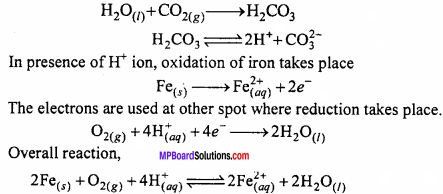Question 4.
Arrange the following metals in the order in which they displace each other from the solution of their salts. Al, Cu, Fe, Mg and Zn. (NCERT)
A metal with lesser standard potential (more reactive) can displace the other metal from solution of its salts.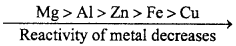Question 5.
Write the definition of Electrochemical cell.
System in which chemical energy is converted to electrical energy by oxidation reduction is known as electrochemical cell or voltaic cell.

Question 6.
What is Electrode potential?
The potential difference developed between the electrodes and electrolyte of an electrolytic cell is known as Electrode potential.

Question 7.
What is a strong electrolyte ? Write two examples.
Electrolyte which completely dissociate in aqueous solution are known as strong electrolyte.
Example : NaCl, KCl, NH4Cl etc.Question 8.
What is meant by standard electrode potential?
Standard electrode potential (E°) of a half cell is the potential difference when one electrode is dipped in molar solution of its ion at 298 K. If electrode is gaseous the pressure of gas must be one atmosphere. In IUPAC system, reduction potential are known as standard electrode potential.

Question 9.
Write Ohm’s law.
According to Ohm’s law, “It states that potential difference across the conductor is directly proportional to the current (I) flowing through it” i.e.,
Mathematically, it can be written as:
I ∝ V
V = IR (R = Resistance, unit = ohm, Q)

Question 10.
What is cell constant?
For a conductivity cell, the ratio of distance between two electrodes (l) and area of cross-section of electrode (A) is called as cell constant.
Cell constant = $$\frac {1}{A}$$ or x = $$\frac {1}{A}$$
Unit of cell constant = cm-1

Question 11.
What is galvanization? Explain.
Iron is coated with the layer of zinc to protect it from rusting. This process is known as galvanization. The galvanized iron articles keep their lustre due to the coating of invisible protective layer of basic zinc carbonate, (ZnCO3) or zinc hydroxide (Zn(OH)2).

Question 12.
Why is it not possible to determine the Electrode potential of a single half cell?
Because Electromotive force of two electrodes containing a complete circuit can be measured.Question 13.
Write the unit of specific conductance.
ohm-1metre-1= Ω-1m-1 = Sm-1

Question 14.
What is the relation between equivalent conductance and specific conductance?
Λceq = $$\frac {1ooo×k}{c}$$

Question 1.
Define conductivity and molar conductivity for the solution of an electrolyte. Discuss their variation with concentration. (NCERT)
Conductivity:
Conductivity of a solution is defined as the conductance of a solution of 1 cm length and having 1 sq. cm as the area of cross-section.

Molar conductivity:
Molar conductivity of a solution at a dilution (V) is the conductance of all the ions produced from one mole of the electrolyte dissolved in V cm3 of the solution when the electrodes are one cm apart and area of cross – section of the electrodes is so large that the whole of the solution is contained between them. It is usually represented by Λm.

Variation with concentration:
The conductivity of a solution (Both for strong and weak electrolytes) decreases with decrease in concentration of the electrolyte i.e., on dilution. This is due to the decrease in the number of ions per unit volume of the solution on dilution. The molar conductivity of a solution increase in the decrease in concentration of the electrolyte i.e., on dilution. This is due to the decrease in the number of ions per unit volume of the solution on dilution.

The molar conductivity of a solution increases with decrease in concentration of the electrolyte. This is because both number of ions as well as mobility of ions increases with dilution. When concentration approaches zero, the molar conductivity is known as limiting molar conductivity.

Question 2.
What is salt bridge ? Write its two functions.
‘U’ shaped tube filled with KCl or KNO3 in Agar – Agar solution or gelatin, is known as salt bridge. It connects the two half cell.

Functions:

1. It allows the flow of current by completing the circuit.
2. It maintains the electrical neutrality.

Question 3.
Write difference between Metallic conduction and Electrolytic conduction.
Differences between Metallic conduction and Electrolytic conduction:
Metallic conduction:

• Metallic conduction takes place by movement of electrons.
• There is no chemical change.
• There is no transfer of matter.
• In metallic conduction conductivity decreases with increase in temperature.

Electrolytic conduction:

• Electrolytic conduction takes place by movement of ions.
• Due to chemical change decomposition of electrolyte takes place.
• Transfer of matter takes place as ions.
• In electrolytic conduction conductivity
• increases with increase in temperature.

Question 4.
What are the difference between emf (Cell potential) and potential difference:
Differences between EMF (Cell potential) and Potential difference
EMF (Cell potential):

• It is the potential difference between the two terminals of the cell when no current is flowing in the circuit, i.e., in an open circuit.
• It is the maximum voltage which can be obtained from a cell.
• It can be measured by potentiaometrie method.
• Work performed by electromotive force is the maximum work done by a cell.
• It is responsible for continuous flow of current in electric circuit.

Potential difference:

• It is the difference of the electrodes potentials of the two electrodes when the cell is sending current through the circuit.
• It is the less than the maximum voltage as it is the difference of electrode potential.
• It can be measured by simple voltmeter also.
• Work performed by potential difference is less than the maximum work done by a cell.
• It is not responsible for the continuous flow of current in circuit.

Question 5.
What is specific conductance? Give its unit.
Specific conductivity:
The reciprocal of resistivity is called specific conductivity. It is defined as the conductance between the opposite faces of one centimeter cube of a conductor. It is denoted by K (kappa).
Thus.
K = $$\frac {1}{ρ}$$ (∵ ρ = $$\frac {RA}{l}$$
K = $$\frac {1}{R}$$ × $$\frac {1}{A}$$
where, R = Resistance, A = Cross – sectional area of electrodes and l = Length between the electrodes.
Unit = K = $$\frac {1}{ohm}$$ × $$\frac { cm }{ { cm }^{ 2 } }$$ = ohm-1 cm-1
S.I. Unit Scnf1 or Ohm-1 cm-1.Question 6.
What is resistivity of any solution?
Resistivity:
When current flow in the solution through two electrodes the resistance is proportional to length and inversely proportional to cross – sectional area A.
R ∝$$\frac {l}{A}$$
or R = ρ$$\frac {l}{A}$$
or ρ = R × $$\frac {A}{l}$$
The constant p (rho) is called resistivity or specific resistance.
Unit: If Z is expressed in cm, A in cm2 and R in ohm, the unit of resistivity will be
$$\frac { c{ m }^{ 2 }\times ohm }{ { cm } }$$ = ohm cm
If l = 1 cm and A = 1 cm2 then ρ = R
or Resistivity of any solution is the resistance of 1 cm cube.

Question 7.
What is equivalent conductance?
Equivalent conductance:
“Conductance of total ion produced by one gram equivalent of electrolyte in the solution is called equivalent conductance.” It is denoted by Λeq.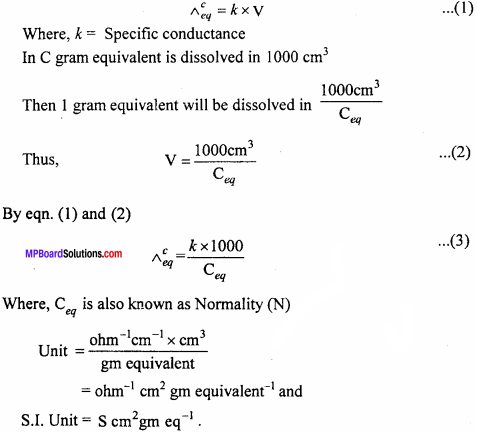Question 8.
What is molar conductance?
Molar Conductivity:
The molar conductivity of a solution at definite concentration of (or dilution) and temperature is the conductivity of that volume which contains one mole of the solute and is placed between two parallel electrodes 1 cm apart and having sufficient area to hold whole of the solution. It is denoted by Λm.
Mathematically,
Λ m=k × V .. (1)
Where, V is the volume in ml in which one gram mole of substance is dissolved.
If M is molarity or m moles are dissolved in 1000 ml.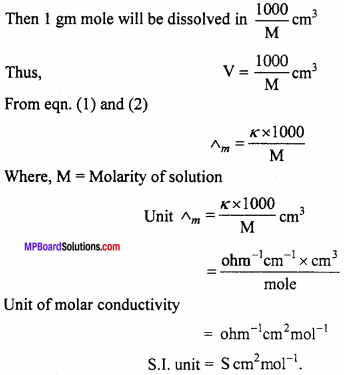Question 9.
Define cell constant. Develop a relation between specific conductance and cetfconstant.
Cell constant:
In any conductive cell, the distance between two electrodes and surface area of .electrode A are constant. The ratio of l and a is called cell constant i.e.
cell constant = $$\frac { l(cm) }{ a{ ({ cm) }^{ 2 } } }$$
Unit of cell constant is cm-1 and it is generally expressed by x.

Relation between specific conductance and cell constant:
For a conductor, the resistance R is directly proportional to length R and inversely proportional to area of crosssection of electrolyte.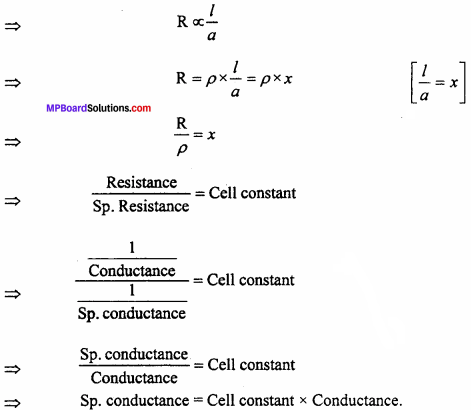Question 10.
What are the factors which influence the electrical conductance of electrolytes?
The main factor which influence the electrical conductivity are following :
1. Temperature : It influence following interactions.

• Interionic attractions : It depends upon the solute-solute interactions. Which is found between the ions of solute.
• Solvation of ions : It depends upon solute-solvent interactions. It is relation between ions of solute and solvent molecules.
• Viscosity of solvent : It depends upon solvent-solvent interactions. Solvent molecules are related with each other.

With increase in temperature all these three effects decrease and average kinetic energy of ions increases. Thus, with increase of temperature, resistance of solution decreases and hence conductance increases.

2. Nature of electrolyte:
The conductance of solution depends upon the nature of electrolyte. On the basis of conductance measurement electrolytes are classified as strong electrolyte and weak electrolyte. Strong electrolytes have high value of conductance even at higher concentration also.

3. Dilution or concentration:
It is main factor which influence electrical conductance. Effect of dilution or concentration can be studied indivisually in equivalent conductance, specific conductance and molar conductance. But for a general concept of electrical conductance of solution as the concentration is lowered or dilution increases, electrical conductance of whole solution increases.

Question 11.
What is an Electrolytic cell and how does it work?
Electrolytic cells:
In these cells electric current is supplied through an external source, as a result of which chemical reactions take place which is called electrolysis like Electrolysis of water, NaCl, Al2O2 etc. For example in Solvay trough cell electrode is immersed in sodium chloride solution and electric current is passed due to which NaCl electrolyses. At mercury cathode sodium is released and at anode chlorine is released. Sodium forms amalgam with mercury and is taken out of the cell.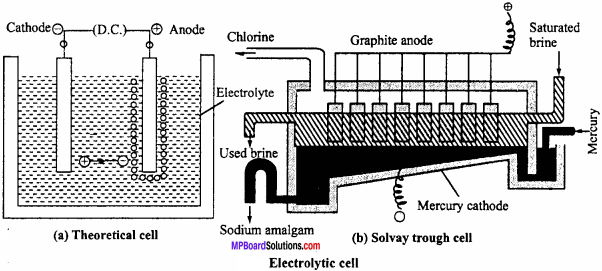Electrolysis : NaCl → Na+ + Cl

At cathode : Na+ + e → Na
Na + Hg → (Na – Hg) Amalgam

At anode : Cl – e → Cl
Cl + Cl → Cl2
In electrolytic cell, electricity is supplied through an external source. Thus, positive pole is anode and negative pole is cathode.Question 12.
What is meant by electromotive force of an electrochemical cell?
The difference in electrode potentials of the two electrodes of an electrochemical cell is known as electromotive force or cell potential. It is expressed in volt. Due to difference in potential electric current flows from an electrode of lower potential to an electrode of higher potential. EMF of the cell can be expressed in terms of reduction potential as :

Cell potential = Standard electrode potential of R.H.S. electrode – Standard electrode potential of L.H.S. electrode
Thus, Ecell = Eredn. (right) – Eredn. (left)
Ecell = Eredn.(cathode) – Eredn.(anode)
EMF of a cell is measured by connecting the voltmeter between the two electrodes of a cell. EMF of a cell depend on the concentration of solutions of both half cells and nature of the two electrodes. For example, In Daniel cell, concentration of CuSO4 and ZnSO4 solutions in the two half cells is 1M and at 298 K EMF of the cell is 1.10 volt.

Question 13.
What is electrochemical series? Write its application.
or, Write application and characteristics of Electrochemical series.
The series in which elements are arranged in increasing order of standard electrode potential is known as electrochemical series.

Applications of Electrochemical Series :

1. Determination of EMF of cell:
EMF of a cell is the difference between standard reduction potential E° of its cathode and anode.
cell = E°cathode – E°anode
If e.m.f. is positive, then the cell reaction proceeds in the required direction and if e.m.f of the cell is negative the cell reaction proceeds in the opposite direction.

2. Calculation of Equilibrium constant of cell:
Equilibrium constant k of the cell can be calculated by determining the e.m.f of the cell by the help of Electrochemical series.
Characteristics:

• Metals which are above hydrogen in the series react with acids to produce hydrogen gas.
• This series represents the standard electrode potential elements i.e, tendency to accept electrons. Elements of negative E° possess the tendency to loose electron.
• Elements which come before in the series displace the metals placed below the series, from their salts.
• At the top of the series strongly reducing elements are present because they possess the tendency to loose electrons.
• Example : Li and below strongly oxidizing like F possess the tendency to accept electron.

Question 14.
What do you understand by standard potential of a half cell? How is the standard potential of a half cell determined?
Standard electrode potential E° of an electrode (half cell) is that value of potential when all the substances are at one atmospheric pressure and activity of the species present in the form of reactant and product is one unit. Standard electrode potential (E°) is measured by the use of potentiometer.
cell = E°R – E°L = E°half cell – E°ref

Question 15.
Differentiate between Electrochemical cell (Galvanic cell) and Electrolytic cell.
Differences between Electrochemical and Electrolytic cells:
Electrochemical cell:

• It is a device to convert chemical energy into electrical energy.
• It consists of two electrodes in different compartments joined by a salt bridge.
• Redox reactions occurring in the cell are spontaneous.
• Free energy decreases with operation of cell, i.e., ∆G <0.
• Useful work is obtained from the cell.
• Anode works as negative and cathode as positive electrodes.
• Electrons released by oxidation process at anode go into external circuit and pass to cathode.
• To set – up this cell, a salt bridge/porous pot is used.

Electrolytic cell:

• It is a device to convert electrical energy into chemical energy.
• Both the electrodes are in same solution.
• Redox reactions occurring in the cell are non – spontaneous.
• Free energy increases with operation of cell, i.e., ∆G >0.
• Work is done on the system.
• Anode is positive and cathode is negative.
• Electrons enter into cathode electrode from external source and leave the cell at anode.
• No salt bridge is used in this cell.

Question 1.
What is standard hydrogen electrode? How is it prepared?
Standard hydrogen electrode:
This consists of gas at 1 atmospheric pressure bubbling over a platinum electrode immersed in 1 M HCl at 25°C (298 K) as shown in figure. The platinum electrode is coated with platinum black to increase its surface. The hydrogen electrode thus constructed forms a half cell which on coupling with any other half cell begins to work on the principle of oxidation or reduction. Electrode depending upon the circumstances works both as anode or cathode.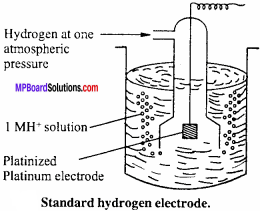Cell reaction of standard hydrogen electrode (SHE) when it acts as anode is
H2(g) → 2H2+ + 2e
It is as represented as
H2(g)(1 atm) Pt |H3O+aq(1.0M)
When it acts as cathode, the cell reaction is
2H+ + 2e → H2(aq)
and it is represented as
H3O+(aq)(1.0M)|(latm)Pt
Standard hydrogen electrode (SHE) is arbitrarily assigned a potential of zero.

Question 2.
Derive Nernst Equation for single electrode potential.
Value of standard electrode potential given in electrochemical series is applicable only when the concentration of electrolyte is 1M and temperature is 298 K. But in electrochemical cells the concentration of electrolyte is not definite and electrode potential depends on concentration and temperature. In such condition single electrode potential can be expressed by Nernst equation.
For a reduction half reaction, Nernst equation can be expressed as follows: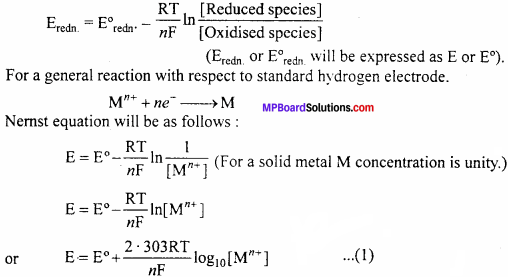Where, E = Reduction electrode potential
E° = Standard electrode potential (Mn++concentration 1M and at 298 K)
R = Gas constant = 831 JK-1mol-1, T = Temperature (in Kelvin) = 298 K
n = Valency of metal ion, F = 1 Faraday (96,500 coulomb)
On substituting the values : E = E°+$$\frac {0.059}{n}$$ log10[M+] … (2)
Equation (2) is Nemst equation for single electrode potential.

Question 3.
Write the Faraday’s laws of electrolysis.
The law states that, “The mass of any substance deposited or liberated at any electrode is directly proportional to the quantity of electricity passed.”
Thus, if W gm of the substance is deposited on passing Q coulomb of electricity, then W ∝Q or W = ZQ
Where, Z is a constant of proportionality and is called electrochemical equivalent of the substance deposited. If a current of I ampere is passed for t second, then Q = I x t. So that,
W = Z x Q = Z x l x t
Thus, if Q = 1 coulomb, I = 1 ampere and t = 1 second, then W = Z. Hence, electrochemical equivalent of a substance may be defined as, “The mass of the substance deposited when a current of one ampere is passed for one second.”
As one Faraday (96500 C) deposits one gram equivalent of the substance, hence electrbchemical equivalent can be calculated from the equivalent mass.
i.e., Z = $$\frac {Equivalent mass of the substance}{96500}$$

It states that, “When the same quantity of electricity is passed through solutions of different electrolytes connected in series, the weight of the substances produced at the electrodes are directly proportional to their equivalent mass.”

For example, for CuSO4 solution and AgNO3 solution connected in series, if the same quantity of electricity is passed, then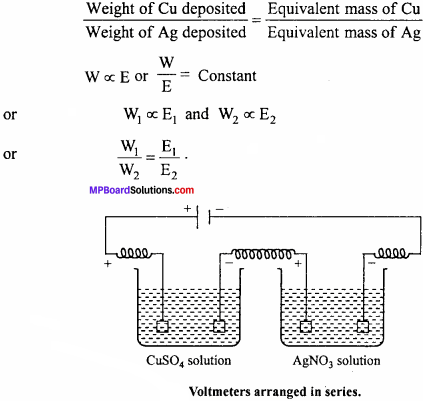Question 4.
What is rusting of iron? Describe Electrochemical theory of rusting. An^Corrosion : Process by which the layers of undesirable compounds are formed on the surface of a metal on its exposure to atmospheric condition are called corrosion. Rusting of iron is an example of corrosion, chemically it is Fe2O3xH2O.

Electrochemical theory of rusting :
Anode reaction:
On one spot of iron sheet, oxidation takes place and this spot behaves as an anode.
Fe(s) → Fe2+(aq) + 2e; E° = + 0.44 V.
The electrons which are released at this spot travel through the metal and reach another spot on the metal which acts as cathode. These electrons cause the reduction of oxygen in the presence of hydrogen ions (H+). H+ ions are formed due to decomposition of carbonic acid formed by dissolution of CO2 in H2O.
H2O + CO2 → H2CO3
H2CO3 ⇌ 2H+ +CO32-+

Cathode reaction:
O2(g) + 4H+(aq) → 2H2O; E° = 1.23V

Overall reaction:
2Fe(s) + O2(g) + 4H+(aq) → 2Fe2+ +2H2O(l); E°cell = 1.67V

Fe2Fe2+ ion formed travel on the surface of article and react with more oxygen in the presence of H2O. This results in the formation of ferric oxide.

4Fe2+aq + O2(g) + 4H2O(l) → 2Fe2O3 + 8H+aq

Water present causes the hydration of ferric oxide formed.
Fe2O3 + XH2O → Fe2O3.xH2O (rust) Thus, rust is formed.Question 5.
What is Kohlrausch law? Give its two applications.
Kohlrausch in 1875 gave a generalisation known as Kohlrausch’s law, “At infinite dilution when the dissociation of the electrolyte is complete, each ion makes a definite contribution towards molar conductance of the electrolyte irrespective of the nature of the other ion with which it is associated.”
Or
“The value of molar conductance at infinite dilution is given by the sum of the contributions of ions (cation and anion).”
Mathematically,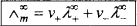Where, λ+ and λ are ionic contributions or ionic conductances of cation and anion while v+ and vare the number of cations and anions in the formula unit of electrolyte.

Applications of Kohlrausch’s law:
(i) Calculation of molar conductance at infinite dilution for weak electrolytes :
Molar conductance or equivalent conductance of weak electrolytes cannot be obtained graphically by extrapolation method, since these are feebly ionized. Kohlrausch’s law enables indirect evaluation in such cases. For example, molar conductances of acetic acid can be obtained from the knowledge of molar conductances at infinite dilution of HCl, CH3COONa and NaCl which are strong electrolytes. From Kohlrausch’s law, it is clear that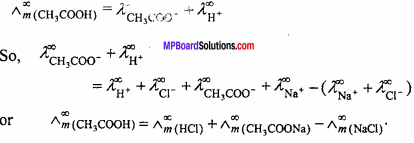(ii) Determination of degree of dissociation: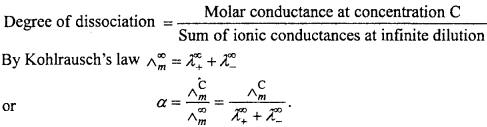Question 6.
Draw a labelled diagram of Daniel cell and explain ceLl reaction.
Or, Draw a labelled diagram of electrochemical cell and write cell reaction.
Electrochemical cell:
In the redox reactions, the transfer of electrons between oxidizing and reducing agents occurs through wire and thus chemical energy changes into electrical energy. The device on which chemical energy changes into electricaL energy is called electrochemical cell. These are also known as galvanic or voltaic cells. Working of these cells can be understood with the example of Daniel cell.Daniel çell:
In this cell, Zn rod is dipped in ZnSO4 solution and Cu rod in copper sulphate solution. Both solutions are connected through KCL salt bridge. When Zn and Cu electrodes are connected by wire and galvanometer, flow of electrons from Zn to Cu occurs. Zinc atoms change into Zn2+ and electrons reach at Cu electrode, where Cu2+ changes into Cu metal and this copper deposits on electrode.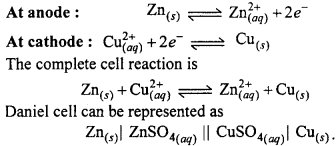Electrochemistry Numerical Questions

Question 1.
Calculate the potential of hydrogen electrode in contact with a solution whose pH is 10. (NCERT)
Solution:
If pH of solution is 10 means its [H+] ion concentration is 10-10 M.
Using, Ecell = E°cell – $$\frac {0.059}{n}$$ log$$\frac { 1 }{ [{ H }^{ + }] }$$
Here E°cell = 0, n =2, [H+] = 10-10
∴Ecell = 0 – $$\frac {0.059}{2}$$ log $$\frac { 1 }{ [{10}^{ -10 }] }$$ = -0.59V

Question 2.
Calculate the standard cell potentials of galvanic cells in which the following reactions take place : (NCERT)
1. 2Cr(s) + 3Cd2+(aq) → Cr3+(aq) + 3Cd
2. Fe2+(aq) +Ag+(aq) → Fe3+(aq) + Ag(s). Calculate the ∆rG°, and equilibrium constant of the reactions.
Solution:
1. E°cell = E°Ecathode – E°Eanodg
= – 0.40 -(-0.74) = +0.34V
∆G° = -nFE° = -6 x 96500 x 0.34
= -196860J = -196.86kJmol-1
∆G° =-2.303 RT log K
– 196860 =-2.307 x 8.314 x 298 log K
or log K = 35.5014 or K
= Antilog 34.5014 = 3.19 x 1034

2. E°cell = E°Ecathode – E°Eanodg
= 0.80 – (0.77) = +0.03V
∆G° = -nFE° = -1 x 96500 x 0.03
= -2895Jmol-1
= – 2-895 kJmol-1
∆G° = -2-303 RT log K
– 2895 Jmol-1 = -2.303 x 8.314 x 298 log K or
or K = Antilog of 0.5074 = 3.22.Question 3.
Using the standard electrode potentials given in Table 3.1 (in your Text-book), predict if the reaction between the following is feasible : (i) Fe3+(aq) and I(aq)
(ii) Ag+(aq) and Cus
(iii) Fe3+(aq) and Br(aq)
(iv) Agsand Fe3+(aq)
(v) Br2(aq) and Fe2+(aq). (NCERT)
A reaction is feasible if EMF of the cell is +ve.
Cathode : At which reduction occurs.
Anode : At which oxidation occurs.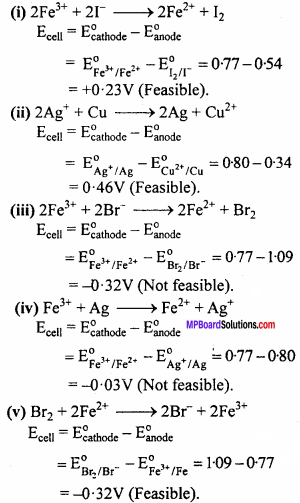Question 4.
If specific conductance of 0.02 mol L-1 KCI solution at 298 K is 2.48 x 10-2-1cm-1, then calculate its molar conductance.
Solution:
K = 2.48 x 10-2 cm-1, C = 0.02 mol L-1
Λm = $$\frac { 1000k }{ { C }_{ m } }$$
= $$\frac { 1000×2.48×{ 10 }^{ -2 } }{ 0.02 }$$
= 124 Scm2 mol-1

Question 5.
What are weak electrolytes? Give one example. Find out molar conductivity of LiBr aqueous solution infinite dilution when joint conductance of Li-1 ion and Br-1 ion are 38.7 Scm2 mol-1 and 78.40 Scm2 mol-1 respectively.
Solution:
Weak electrolytes : These are the substances which dissociate only to a small extent.
Examples: CH3COOH,NH4OH
Λ LiBr = Λ Li+ + Λ Br
Given that,
Λ Li+ = 38.7Scm2 mol-1
Λ Br = 78.40 Scm2 mol-1
Λ LiBr = 38.7 + 78.40
Λ LiBr = 117.10Scm2mol-1Question 6.
What are strong electrolytes? Find out the molar conductivity of aqueous solution of BaCl2 at infinite dilution when ionic conductance of Ba+2 ion and Cl ion are 127.30 Scm2 mol-1 and 76.34 Scm2 mol-1 respectively.
Solution:
Strong electrolytes : These are substances which dissociate almost completely into ions under all dilutions.
Examples : NaCl,HCl,CH3COONa
Λ BaCl2 = Λ Ba2+ + 2 Λ Cl
Given that,
Λ = 127.30Scm2+mol-1
Λ Cl-1 = 76.34Scm2+mol-1
Λ BaCl2 =127.30+2(76.34)
= 127.30 + 152.68
= 279.98 Scm2+mol-1

MP Board Class 12th Chemistry Important Questions